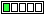All about flooble | fun stuff | Get a free chatterbox | Free JavaScript | Avatarsperplexus dot infoDefining a triangle. (Posted on 2021-03-06)The area of a triangle ABC is 5cm2.
AB=2cm and BC=5cm.

How much is AC?Comments: ( Back to comment list | You must be logged in to post comments.)re: Solution| Comment 2 of 3 |(In reply to Solution by Kenny M)

While perhaps trivial, this occurred to me: given a and b and Area and then asked to find c would have yielded two answers, had not Area = 1/2 a b. That the area is maximized with a right angle, and so gives a single answer, is proven by differentiation:

Area = 1/2 a b sin_theta
d_Area/d_sin_theta = 0
gives theta = 90.

For smaller Areas, the two sin_theta solutions are the acute and obtuse angles on either side of 90, and had Area been greater than 1/2 a b, there'd be no answer at all.

Edited on March 6, 2021, 6:27 pm
 Posted by Steven Lord on 2021-03-06 15:56:46Please log in:

 Search: Search body:
Forums (0)